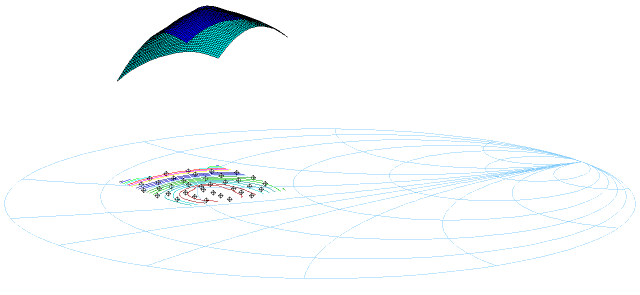# Surface Interpolation for Scilab

use plot3d() and contour() with scattered data

## Presentation

Here is a new toolbox for Scilab. This toolbox enables the use of plot3d() and contour() functions even if your data are not located on a regular grid.
Indeed, a new function interpolates your data and generates automatically a regular grid. This is well suited for load-pull analysis (this example).
This toolbox includes 2 functions :
• plotsmith(color) : display the smithchart, even for 3D applications.

• interpolate_grid(data,scale_factor) : generate a 3 elements list. The fist element is the X vector, the second one is the Y vector and the third one is the Z matrix of the interpolated data. Data is a matrix : each column has to contain X,Y and Z data points (real only).The scaling factor is the ratio beetween the interpolated grid and the smallest vector in your data.

## Example

// This exemple displays the picture below
M=fscanfMat("C:\exemple.txt");
M(:,3)=M(:,3)./1000 // Unit change from mW to W
clf() ;
plotsmith(12);
plot2d(M(:,1),M(:,2),-8);
new_data=interpolate_grid(M,10);
contour(new_data(1),new_data(2),new_data(3),20);
plot3d(new_data(1),new_data(2),new_data(3),alpha=28,theta=236);This new function generates a regular grid from scattered input data